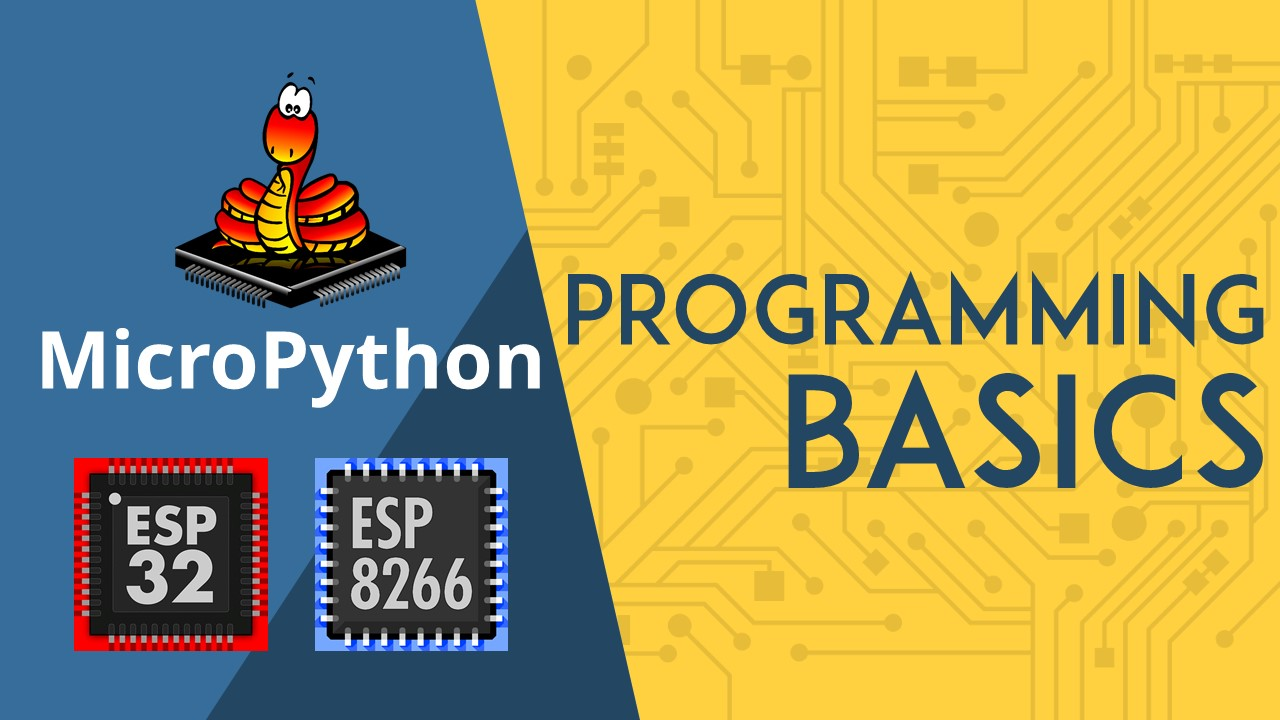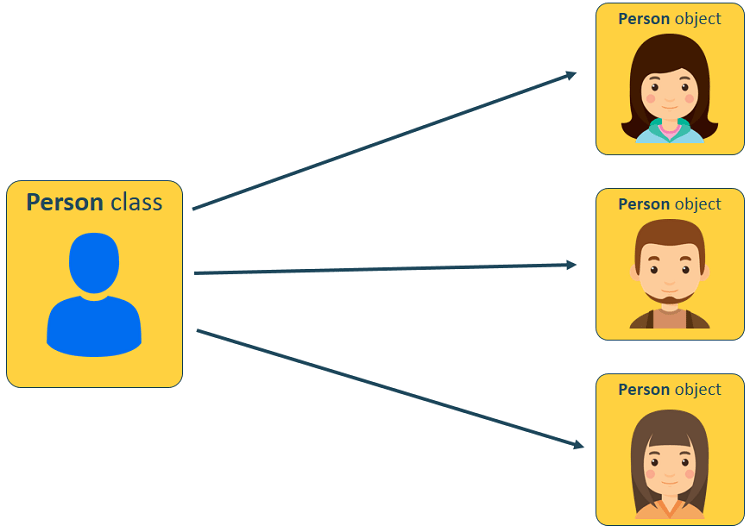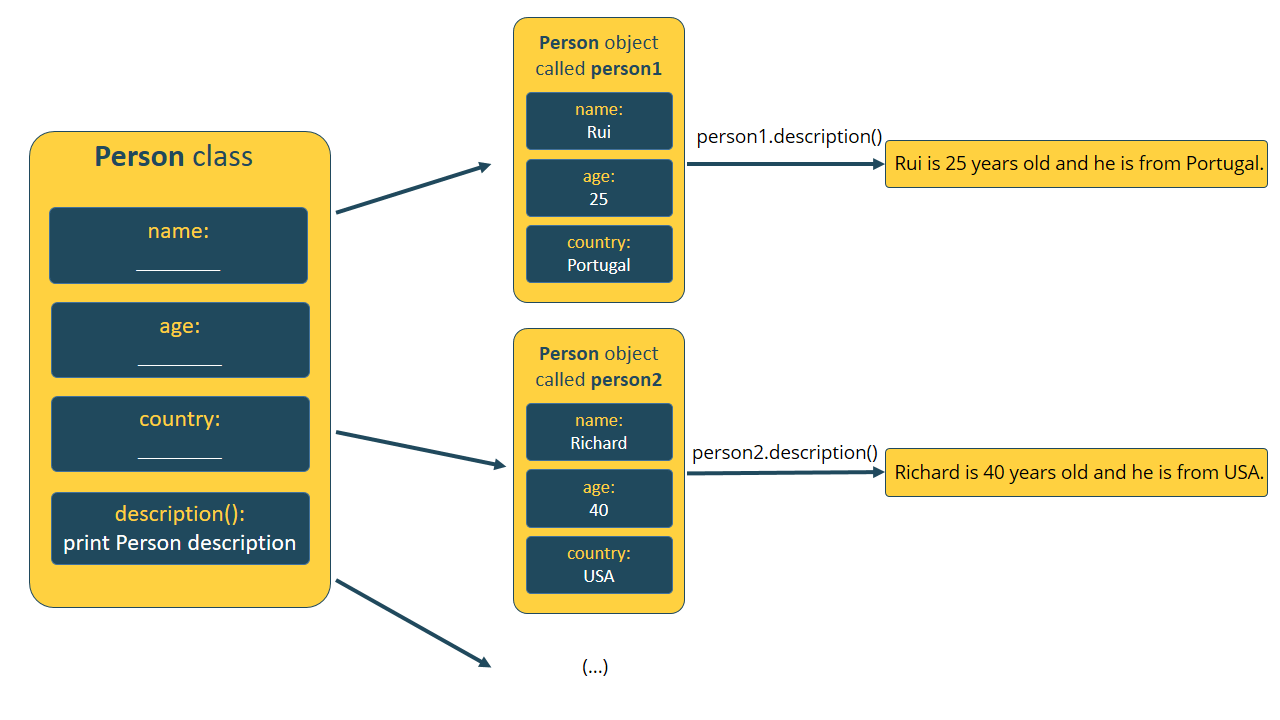# MicroPython Programming Basics with ESP32 and ESP8266

MicroPython is a re-implementation of Python programming language targeted for microcontrollers and embedded systems like the ESP32 or ESP8266.

Programming in MicroPython is very similar to programming in Python: all of the language features of Python are also in MicroPython, apart from a few exceptions. Because microcontrollers and embedded systems are much more limited than our computers, MicroPython does not come with the full standard library by default.If you already know how to program in Python, programming in MicroPython is the same. You just need to bear in mind that MicroPython is used for constrained devices. So, you must keep your code as simple as possible.

This article explains the basics of Python programming language syntax that also apply to MicroPython, like:

• Mathematical operators
• Relational operators
• Data types
• print() function
• Conditional statements
• While and for loops
• User defined functions
• Classes and objects
• Modules

## Prerequisites

In this tutorial we’re going to use uPyCraft IDE as a development environment, but you can use any other program. To install and get started with uPyCraft IDE, follow these next tutorials:

## Mathematical Operators

Micropython can perform mathematical operations. The following table shows the mathematical operators supported:

 Operator Mathematical Operation + Addition – Subtraction * Multiplication / Division // Division, discarding the decimal point % Remainder after division

In the Shell, try several operations to see how it works. For example:

```>>> 2+2*9-3
17
>>> 28594/2312
12.36765
>>> 214522236/7.5
2.860297e+07
>>> 23//2
11
>>> 25%3
1
```

You can perform other mathematical operations if you import the math module, like square root, trigonometric functions, logarithm, exponentiation, etc..

## Relational Operators

You can make comparisons using relational operators. These compare the values on either sides and show the relation between them.

 Operator Description == Equal to != Not equal to > Greater than < Less than >= Greater than or equal to <= Less than or equal to

Try several comparisons and test the result:

```>>> 2 == 3
False
>>> 4 == 4
True
>>> 3 > 2
True
>>> 489808234 != 2223
True
>>> 4.5 >= 4.5
True```

## Assigning Values to Variables

In Python you don’t need to declare what type each variable is. If you’re used to program your boards using Arduino IDE, you know that you need to declare the type of a variable when creating a new variable. There isn’t such thing in Python.

Variables are simply a storage placeholder for values: number or text. To assign a value to a variable you use the equal sign (=), with the variable name on the left and the value on the right.

For example, to create a variable to hold the GPIO number where an LED is connected to, you can simply type the following:

`led_pin = 23`

In the Arduino IDE, you would have something like:

`const int led_pin = 23;`

As you can see, Python is much simpler than programming in C (in Arduino IDE).

Note: the names you give variables can’t have spaces and are case sensitive, so led_pin is different from LED_PIN or Led_Pin.

## Data Types

Variables can store several types of values, not just whole numbers. That’s where data types come in. A data type is a classification of a value that tells what operations can be done with the value and how it should be stored.

The following table shows the data types we’ll use most often in our projects.

 Data type Description int (Int) Integer (whole number) float (Float) Number with a decimal point str (String) Set of characters between quotation marks bool (Boolean) True or False

Let’s create variables with different data types:

```>>> a = 6
>>> b = 95.32
>>> c = 'Hello World!'
>>> d = True```
• The first value assigned to a, is an integer, which is a whole number.
• The b variable contains a float value, which is a number with a decimal.
• The third value, ‘Hello World!’, is a string, which is a series of characters. A string must be put inside single (‘Hello World!’) or double quotation (“Hello World!”) marks.
• Finally, d is a Boolean, which is a type that can only take either True or False.

There is a function to check the data type of a variable: the type() function. This function accepts as argument the variable you want to check the data type.

`type(variable)`

For example, after declaring the variables in the previous example a, b, c, and d, you can check its data type. For example, if you type:

`>>> type(a)`

It returns:

`<class 'int'>`

This tells that a is an int (integer). Experiment with the other variables and you should get:

```>>> type(b)
<class 'float'>
>>> type(c)
<class 'str'>
>>> type(d)
<class 'bool'>```

## print() Function

The print() function prints the message between parentheses into the Shell. This is specially useful in our projects to debug the code and keep track of what’s going on. For example:

```>>> print('LED is on')
LED is on```

Comments in Python start with the hash character (#) and continue to the end of the line. A comment is useful to add “notes” in your program or to tell anyone who reads the program what the script does. This doesn’t add any functionality to your program. For example:

`# This is just a comment`

Because in MicroPython we are working under constrained conditions, there are occasions in which you should avoid adding comments to save space on the ESP memory.

## Conditional Statements

To write useful programs, you’ll probably need to perform different actions depending on whether a certain condition is True or False. We’re talking about conditional statements. They have the following structure:

```if <expr1>:
<statement1>
elif <expr2>:
<statement2>
elif <expr3>:
<statement3>
(...)
else:
<statementn>```

<expr> is a Boolean expression and it can be either True or False. If it is True, the <statement> right after it is executed. The <statement> should be indented so that Python knows what statement belongs to each expression.

The elif statement stands for else if and runs only if the first if condition is not True.

The else statement only runs if none of the other expressions are True.

There’s no limit to the number of elif statements in a program. It’s also not necessary to include an else clause, but if there is one, it must come at the end.

In the Arduino IDE, we use {} curly brackets to define code blocks. With MicroPython, we use indentation. Additionally, you need to use a colon : after each expression. Contrary to the Arduino IDE, the expression doesn’t need to be inside parentheses.

Important: Python’s standard indentation is 4 spaces. In MicroPython indentation should be only 2 spaces to fit more code into the microcontroller memory.

## While and For loops

Loops allow you to execute a block of code multiple times for as long as a condition is met. There are two kinds of loops: while and for loops. For example, you can print all numbers from 1 to 10 with a while loop:

```number = 1
while number <= 10:
print(number)
number = number + 1```

The code that belongs to the while loop, indicated by the indentation, is executed as long as the value in the number variable is less than or equal to (<=) 10. In every loop, the current number is printed and then 1 is added to it.

You can also print numbers from 1 to 10 using a for loop, like this:

```number = 1
for number in range(1, 11):
print(number)```

The for loop is executed as long as the value in the number variable is within the range of 1 and 11. The range() function automatically assigns the next value to the number variable, until 1 below the final number you specify.

You should use a for loop when you want to repeat a block of code a certain number of times. Use a while loop when you want to repeat code until a certain condition is no longer met. In some situations, you can use either one, oftentimes one is more suitable than the other.

Similar to the conditional statements, the for and while Boolean expressions should have a colon : right after them, and the expressions to be executed should be indented.

## User-defined Functions

To define a new function, you use the word def followed by the name you want to give the function and a set of parentheses (and arguments inside, if necessary). After the parentheses you add a colon : and then tell the function what instructions to perform. The statements should be indented with 2 spaces (in MicroPython). For example:

```def my_function(<arg1>, <arg2>, ...):
<statement>
(...)
return```

For example, a function that converts the temperature in Celsius to Fahrenheit could be the following:

```def celsius_to_fahrenheit(temp_celsius):
temp_fahrenheit = temp_celsius * (9/5) + 32
return temp_fahrenheit```

The celsius_to_fahrenheit() function accepts as argument a temperature in Celsius (temp_celsius). Then, it does the calculation to convert the temperature. Finally, it returns the temperature in Fahrenheit (temp_fahrenheit).

Note: functions don’t necessarily need to return something. They could just perform some work without the need to return anything.

## Classes and Objects

Python is an Object-Oriented Programming (OOP) language. There are two important concepts you need to understand about OOP: classes and objects.

A class is a blueprint for objects. It defines a set of attributes (data and functions) that characterize an object. The functions inside of a class are called methods. Classes are defined by the keyword class followed by the name of the class. For example:

```class MyClass:
(...)```

Note: by convention, classes’ names in Python should be CapWords. However, you can give whatever name you want.

An object is an instance of a class. It’s simply a collection of data and methods into a single entity. Through the object, you can use all functionalities of its class. Confused? Let’s take a look at a simple example.

If we would like to define several persons in a Python program using the same attributes, we can think of the term person as a class. We may want to define a person using attributes like name, age, country, etc.So, we can create a class called Person. Our class will have the following attributes: name, age, and country. You can add as many attributes as you want. We’ll also create a function (method) that prints a description of the person based on its attributes:

```class Person:
name = ""
age = 0
country = ""
def description(self):
print("%s is %d years old and he is from %s." %(self.name, self.age, self.country))```

As you can see, we define a new class by using the keyword class, followed by the name we want to give to the class.

Inside the Person class, we define several variables to hold values. By default the name and country are empty strings, and the age is 0. Then, we also define a function (method) that prints all the variables values into the Shell.

All functions inside a class should have the self parameter as argument and other arguments, if needed.

The self  parameter refers to the object itself. It is used to access variables that belong to the class. For example, to access the name variable inside the class, we should use self.name.

Now that we’ve create a class, we can create as many Person objects as we want by using that class. The Person object will have a name, age, and country. We’ll also be able to print its description using the description() method.

For example, to create a new Person object called person1:

`>>> person1 = Person()`

Set the object’s properties

To set the name, age, and country of the person1 object. You can do it as follows:

```>>> person1.name = "Rui"
>>> person1.age = 25
>>> person1.country = "Portugal"```

Calling methods

Later in your code you can use the created description() method on any Person object. To call the description() method on the person1 object:

`>>> person1.description()`

This should print the following:

`Rui is 25 years old and he is from Portugal.`

You should now understand that you can create as many objects as you want using the same class and you are able to use the available methods with all the objects of that class.The constructor method

Instead of having to define a class, and then set the object’s properties, which can be time consuming, you can use the constructor method inside your class.

The constructor method is used to initiate data as soon as an object of a class is instantiated. The constructor method is also known as __init__ method.  Using the __init__ method, the Person() class looks as follows:

```class Person():
def __init__(self, name, age, country):
self.name = name
self.age = age
self.country = country
def description(self):
print("%s is %d years old and he is from %s." %(self.name, self.age, self.country))```

Then, to instantiate a Person object with the same attributes we’ve defined earlier, we just need to do the following:

`>>> person1 = Person("Rui", 25, "Portugal")`

If you call the description() on the person1 object, you’ll get the same result:

```>>> person1.description()
Rui is 25 years old and he is from Portugal.```

## Modules

A module is a file that contains a set of classes and functions you can use in your code – you can also call it library. To access the classes and functions inside that code, you just need to import that module into your code.

You can create your own modules, or use already created modules from the standard Python library. When it comes to MicroPython, it only comes with a small subset of the standard Python library, but it does come with a set of modules to control GPIOs, make networks connections and much more.

Importing modules/libraries is as simple as using:

`import module_name`

For example, to import the machine library that contains classes to control GPIOs, type the following:

`import machine`

In most programs you won’t need all the classes from one module. You may just want to import a single class. For example, to import only the Pin class from the machine module:

`from machine import Pin`

## Wrapping Up

In this tutorial we’ve just scratched the surface of Python basics that also apply to MicroPython. If you are used to program electronics using the Arduino IDE, you’ll find that MicroPython has a much simpler and user-friendly syntax. Let’s just summarize some of the main differences between your Arduino sketches and programs in MicroPython:

• You don’t use semicolon ; at the end of a statement
• After Boolean expressions in conditional statements and loops, you use a colon :
• To define code blocks use indentation instead of curly brackets {}
• When creating a variable, you don’t need to define which data type it is – you don’t need to declare a variable
• Indentation in MicroPython is 2 spaces

Recommend reading: MicroPython Programming with ESP32 and ESP8266 eBook

We hope you’ve found this article about the MicroPython basics syntax useful. We’ll be adding tutorials about programming the ESP32 and ESP8266 using MicroPython very soon. So, stay tuned and subscribe the RNT blog!#### [eBook] MicroPython Programming with ESP32 and ESP8266

Learn how to program and build projects with the ESP32 and ESP8266 using MicroPython firmware DOWNLOAD »

Learn how to program and build projects with the ESP32 and ESP8266 using MicroPython firmware DOWNLOAD »

### Recommended Resources

Arduino Step-by-Step Projects » Build 25 Arduino projects with our course, even with no prior experience!

### 10 thoughts on “MicroPython Programming Basics with ESP32 and ESP8266”

1.Nice work

•Thanks 🙂

2.Hi,

Can we install Tensorflow in in ESP32 or esp8266 ?

Would be interested if you can make any tutorial when you get a chance…

Thx

•Hi Raj.
We’re not planning adding any tutorials about Tensorflow in a near future.
Regards,
Sara 🙂

3.Wonderfully written, easy to understand step by step introduction of the basics.

What I would of liked to have seen is an example of actually creating a small module (Library).

•Hi.
This is just a quick getting started guide, so we just included the most basic and essential stuff.
Creating a module is indeed something really interesting. Maybe we’ll add a new tutorial about that in a near future.
Regards,
Sara 🙂

4.Perfektne, praktické, jednoduché.
MicroPython ma svoju budúcnosť a aj ESP32.
Dakujem.
ps: pošlem na cafe.

•Hi Dusan.
You are right!
Thank you so much for supporting our work.
(Ďakujeme vám za podporu našej práce) (used Google translator).
Regards,
Sara

5.